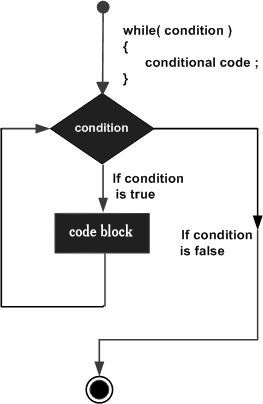# C++ while loop

A while loop statement repeatedly executes a target statement as long as a given condition is true.

## Syntax

The syntax of a while loop in C++ is −

```while(condition) {
statement(s);
}
```

Here, statement(s) may be a single statement or a block of statements. The condition may be any expression, and true is any non-zero value. The loop iterates while the condition is true.

When the condition becomes false, program control passes to the line immediately following the loop.

## Flow DiagramHere, key point of the while loop is that the loop might not ever run. When the condition is tested and the result is false, the loop body will be skipped and the first statement after the while loop will be executed.

## Example

```#include <iostream>
using namespace std;

int main () {
// Local variable declaration:
int a = 10;

// while loop execution
while( a < 20 ) {
cout << "value of a: " << a << endl;
a++;
}

return 0;
}
```

When the above code is compiled and executed, it produces the following result −

```value of a: 10
value of a: 11
value of a: 12
value of a: 13
value of a: 14
value of a: 15
value of a: 16
value of a: 17
value of a: 18
value of a: 19
```
cpp_loop_types.htm